7-3 GMM Application: Classification

[chinese][all]

We can also use GMM to construct a classifier for pattern recognition. Such a GMM-based classifier is denoted as GMMC for short. The construction and evaluation of a GMMC are explained next.

1. At the training stage, we need to obtain a GMM for each class. In other words, we need to use the data of a class to train a GMM. This is done sequentially for each class; there is no interactions between GMM of different classes.
2. At the application stage, we need to send the unknown-class data to the GMM of each class. The predicted class is the one associated with the GMM with the maximum probability.

The following example demonstrates the use of GMM for classification of iris dataset:

Example 1: gmmcIris01.m% Use GMM for the classification of iris dataset % ====== Collect data [DS, TS]=prData('iris'); classLabel=unique(DS.output); classNum=length(classLabel); gmmOpt=gmmTrain('defaultOpt'); % ====== Train a GMM for each class clear data logLike for i=1:classNum fprintf('Training GMM for class %d...\n', i); index=find(DS.output==classLabel(i)); data{i}=DS.input(:, index); [class(i).gmmPrm, logLike{i}] = gmmTrain(data{i}, gmmOpt); end % ====== Compute inside-test recognition rate clear outputLogLike; for i=1:classNum outputLogLike(i,:)=gmmEval(DS.input, class(i).gmmPrm); end [maxValue, computedOutput]=max(outputLogLike); recogRate1=sum(DS.output==computedOutput)/length(DS.output); fprintf('Inside-test recog. rate = %g%%\n', recogRate1*100); % ====== Compute outside-test recognition rate clear outputLogLike for i=1:classNum outputLogLike(i,:)=gmmEval(TS.input, class(i).gmmPrm); end [maxValue, computedOutput]=max(outputLogLike); recogRate2=sum(TS.output==computedOutput)/length(TS.output); fprintf('Outside-test recog. rate = %g%%\n', recogRate2*100);Training GMM for class 1... Training GMM for class 2... Training GMM for class 3... Inside-test recog. rate = 97.3333% Outside-test recog. rate = 97.3333%

In the above example, we partitioned the dataset into a design set (DS) and a test set (TS). We used DS for training and TS for test. Each class is model as a two-Gaussian GMM. The obtained recognition rates are 100% and 96% for inside and outside tests, respectively.

Since the above procedure of training a GMM classifier and evaluating the classifier is used quite often, we can simplify the procedure by using the following two functions:

• gmmcTrain.m: For training a GMM classifier.
• gmmcEval.m: For evaluating a GMM classifier.
In particular, gmmcTrain.m will genearte a set of parameters for GMM classifier (including the prior information), and gmmcEval.m can take the parameters and evaluate the classifier based on a given new set of data. The use of these two functions is shown in the next example.

Example 2: gmmcIris02.m% Use GMM for the classification of iris dataset [DS, TS]=prData('iris'); gmmOpt=gmmTrain('defaultOpt'); gmmcPrm=gmmcTrain(DS, gmmOpt); computedOutput=gmmcEval(DS, gmmcPrm); recogRate1=sum(DS.output==computedOutput)/length(DS.output); fprintf('Inside-test recog. rate = %g%%\n', recogRate1*100); computedOutput=gmmcEval(TS, gmmcPrm); recogRate2=sum(TS.output==computedOutput)/length(TS.output); fprintf('Outside-test recog. rate = %g%%\n', recogRate2*100);Inside-test recog. rate = 97.3333% Outside-test recog. rate = 97.3333%

If we want to visualize the decision boundary imposed by a GMM classifier, we can try the following example which apply GMMC to a nonlinear separable dataset:

Example 3: gmmcNonlinearSeparable01.mDS=prData('nonlinearSeparable'); gmmcOpt=gmmcTrain('defaultOpt'); gmmcOpt.arch.gaussianNum=3; gmmcPrm=gmmcTrain(DS, gmmcOpt); computed=gmmcEval(DS, gmmcPrm); DS.hitIndex=find(computed==DS.output); % This is used in gmmcPlot. gmmcPlot(DS, gmmcPrm, 'decBoundary');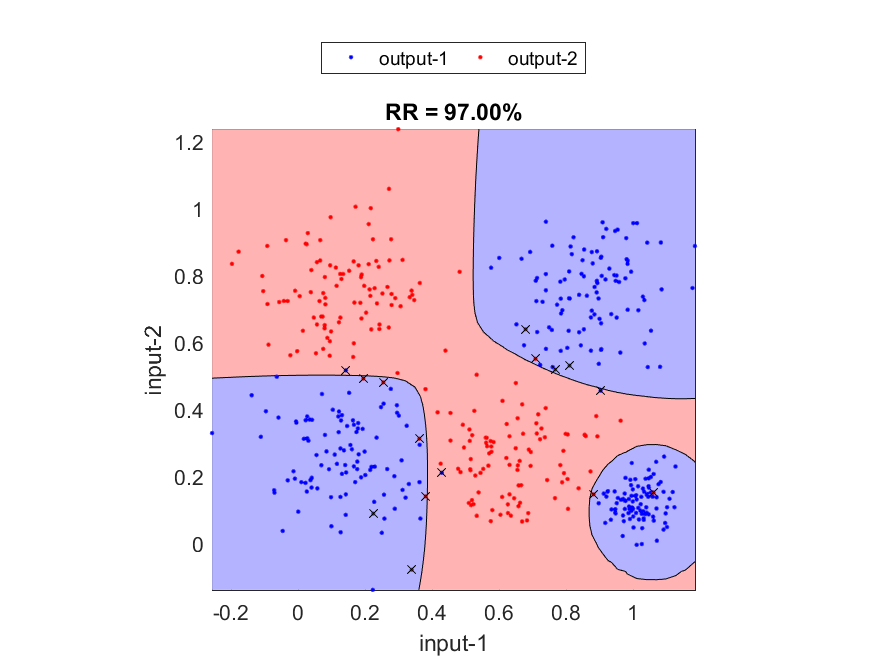In the above example, we set the number of Gaussians to be 3 since we already know the data distribution and 3 is a good choice since class 1 is segmented into 3 disjoint regions. In practice, we are usually dealing with high-dimensional data and such information does not come by easily. As a result, we usually have to resort to trials and errors, as explained later.

The next example demonstrates the PDFs of GMMs associated with these two classes. Due to the combination of several Gaussian PDFs, we are able to model more complicated PDFs of this problem.

Example 4: gmmcNonlinearSeparable02.mDS=prData('nonlinearSeparable'); gmmcOpt=gmmcTrain('defaultOpt'); gmmcOpt.arch.gaussianNum=3; gmmcPrm=gmmcTrain(DS, gmmcOpt); gmmcPlot(DS, gmmcPrm, '2dPdf');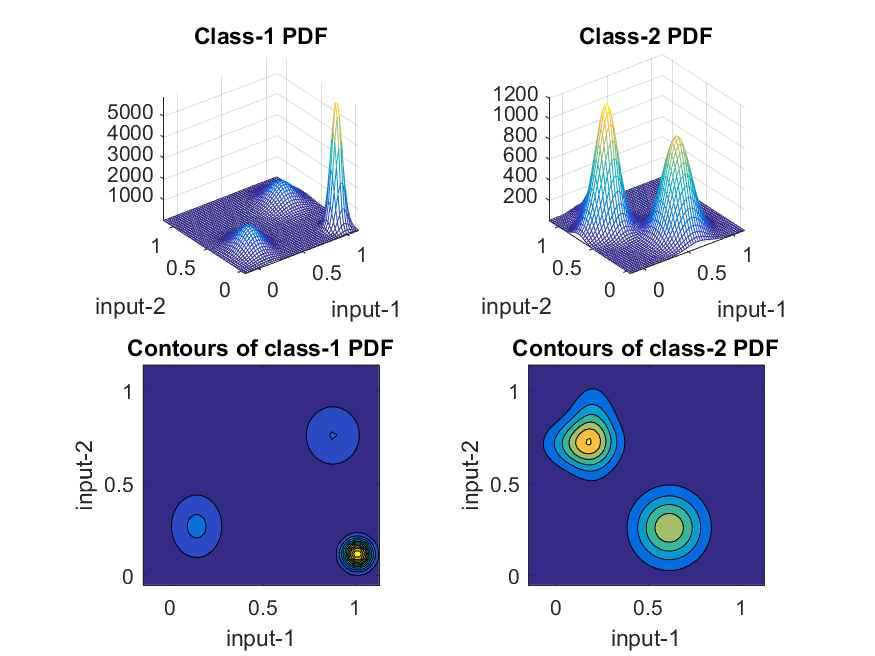Hint
If we set the number of Gaussians to 2 in the above two examples, what would you expect to see? Guess it before you try it. Then try it, you might be amazed at how versatile & flexible the GMMC is!

As mentioned earlier, the performance of GMM is highly related to its number of Gaussian components. In the following example, we can plot the relationship between the recognition rate and GMM's number of Gaussian components.

Example 5: gmmcIris03.m% Use GMM for the classification of iris dataset. % We vary the number of mixtures to get the relationship between recognition rate and number of mixtures of GMM. % ====== Get the dataset [DS, TS]=prData('iris'); classNum=length(unique(DS.output)); gaussianNum=1:16; gmmOpt=gmmTrain('defaultOpt'); trialNum=length(gaussianNum); % ====== Perform training and compute recognition rates recogRate1=[]; recogRate2=[]; for j=1:trialNum fprintf('%d/%d: ', j, trialNum); % ====== Training GMM model for each class for i=1:classNum % fprintf('Training class %d: ', i); index=find(DS.output==i); data{i}=DS.input(:, index); gmmOpt.arch.gaussianNum=gaussianNum(j); [class(i).gmmPrm, logLike{i}]=gmmTrain(data{i}, gmmOpt); end gmmcPrm.class=class; gmmcPrm.prior=dsClassSize(DS); gmmcPrm.task='Iris Classification'; % ====== Compute inside-test recognition rate computedOutput=gmmcEval(DS, gmmcPrm); recogRate1(j)=sum(DS.output==computedOutput)/length(DS.output); % ====== Compute outside-test recognition rate computedOutput=gmmcEval(TS, gmmcPrm); recogRate2(j)=sum(TS.output==computedOutput)/length(TS.output); fprintf('Recog. rate: inside test = %g%%, outside test = %g%%\n', recogRate1(j)*100, recogRate2(j)*100); end % ====== Plot the result plot(gaussianNum, recogRate1*100, 'o-', gaussianNum, recogRate2*100, 'square-'); grid on legend('Inside test', 'Outside test', 4); xlabel('No. of Gaussian mixtures'); ylabel('Recognition Rates (%)');1/16: Recog. rate: inside test = 96%, outside test = 94.6667% 2/16: Recog. rate: inside test = 97.3333%, outside test = 97.3333% 3/16: Recog. rate: inside test = 97.3333%, outside test = 94.6667% 4/16: Recog. rate: inside test = 97.3333%, outside test = 96% 5/16: Recog. rate: inside test = 98.6667%, outside test = 94.6667% 6/16: Recog. rate: inside test = 100%, outside test = 94.6667% 7/16: Recog. rate: inside test = 100%, outside test = 93.3333% 8/16: Recog. rate: inside test = 97.3333%, outside test = 89.3333% 9/16: Recog. rate: inside test = 100%, outside test = 93.3333% 10/16: Recog. rate: inside test = 100%, outside test = 90.6667% 11/16: Recog. rate: inside test = 100%, outside test = 93.3333% 12/16: Recog. rate: inside test = 100%, outside test = 82.6667% 13/16: Recog. rate: inside test = 100%, outside test = 90.6667% 14/16: Recog. rate: inside test = 100%, outside test = 90.6667% 15/16: Recog. rate: inside test = 100%, outside test = 81.3333% 16/16: Recog. rate: inside test = 100%, outside test = 68% [Warning: Using an integer to specify the legend location is not supported. Specify the legend location with respect to the axes using the 'Location' parameter.] [> In <a href="matlab:matlab.internal.language.introspective.errorDocCallback('legend>process_inputs', 'C:\Program Files\MATLAB\R2016a\toolbox\matlab\scribe\legend.p', 499)" style="font-weight:bold">legend>process_inputs</a> (<a href="matlab: opentoline('C:\Program Files\MATLAB\R2016a\toolbox\matlab\scribe\legend.p',499,0)">line 499</a>) In <a href="matlab:matlab.internal.language.introspective.errorDocCallback('legend>make_legend', 'C:\Program Files\MATLAB\R2016a\toolbox\matlab\scribe\legend.p', 303)" style="font-weight:bold">legend>make_legend</a> (<a href="matlab: opentoline('C:\Program Files\MATLAB\R2016a\toolbox\matlab\scribe\legend.p',303,0)">line 303</a>) In <a href="matlab:matlab.internal.language.introspective.errorDocCallback('legend', 'C:\Program Files\MATLAB\R2016a\toolbox\matlab\scribe\legend.p', 254)" style="font-weight:bold">legend</a> (<a href="matlab: opentoline('C:\Program Files\MATLAB\R2016a\toolbox\matlab\scribe\legend.p',254,0)">line 254</a>) In <a href="matlab:matlab.internal.language.introspective.errorDocCallback('gmmcIris03', 'd:\users\jang\books\dcpr\example\gmmcIris03.m', 35)" style="font-weight:bold">gmmcIris03</a> (<a href="matlab: opentoline('d:\users\jang\books\dcpr\example\gmmcIris03.m',35,0)">line 35</a>) In <a href="matlab:matlab.internal.language.introspective.errorDocCallback('goWriteOutputFile>dummyFunction', 'd:\users\jang\books\goWriteOutputFile.m', 85)" style="font-weight:bold">goWriteOutputFile>dummyFunction</a> (<a href="matlab: opentoline('d:\users\jang\books\goWriteOutputFile.m',85,0)">line 85</a>) In <a href="matlab:matlab.internal.language.introspective.errorDocCallback('goWriteOutputFile', 'd:\users\jang\books\goWriteOutputFile.m', 55)" style="font-weight:bold">goWriteOutputFile</a> (<a href="matlab: opentoline('d:\users\jang\books\goWriteOutputFile.m',55,0)">line 55</a>)]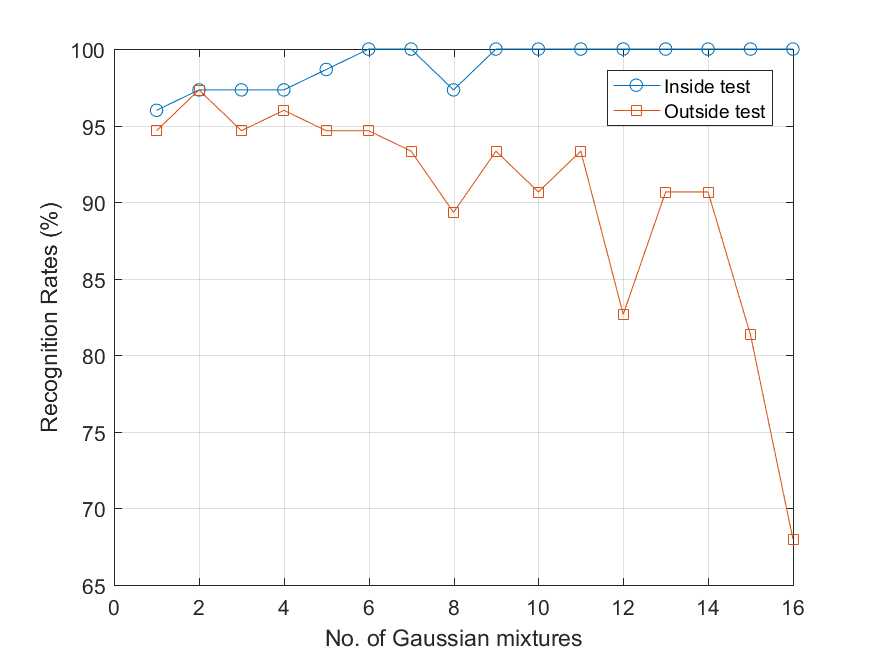The number of Gaussians in GMM represents the flexibility of a GMM. In the above example, we can observe a common characteristics of a classifier:
1. When the classifier have more and more tunable parameters, the inside-test recognition rate will go up all the way, while the outside-test recognition rate will go up initially and then fall down eventually.
2. The optimum configuration of a classifier is usually chosen as the one that can have the maximum outside-test recognition rate.

Since it is a common practice to observe the training and test recognition rates with respective to the number of Gaussians, we have create a function gmmcGaussianNumEstimate.m to accomplish the task. In the following example, we use the wine dataset for such task:

Example 6: gmmcWine02.m[DS, TS]=prData('wine'); count1=dsClassSize(DS); count2=dsClassSize(TS); gmmcOpt=gmmTrain('defaultOpt'); gmmcOpt.arch.gaussianNum=1:min([count1, count2]); plotOpt=1; [gmmData, recogRate1, recogRate2]=gmmcGaussianNumEstimate(DS, TS, gmmcOpt, plotOpt); DS data count = 89, TS data count = 89 DS class data count = [29 36 24] TS class data count = [30 35 24] 1/24: No. of Gaussian = [1;1;1] ===> inside RR = 97.7528%, outside RR = 98.8764% 2/24: No. of Gaussian = [2;2;2] ===> inside RR = 98.8764%, outside RR = 98.8764% 3/24: No. of Gaussian = [3;3;3] ===> inside RR = 100%, outside RR = 100% 4/24: No. of Gaussian = [4;4;4] ===> inside RR = 100%, outside RR = 98.8764% 5/24: No. of Gaussian = [5;5;5] ===> inside RR = 100%, outside RR = 96.6292% 6/24: No. of Gaussian = [6;6;6] ===> inside RR = 100%, outside RR = 97.7528% 7/24: No. of Gaussian = [7;7;7] ===> inside RR = 100%, outside RR = 97.7528% 8/24: No. of Gaussian = [8;8;8] ===> inside RR = 100%, outside RR = 93.2584% 9/24: No. of Gaussian = [9;9;9] ===> inside RR = 100%, outside RR = 94.382% 10/24: No. of Gaussian = [10;10;10] ===> inside RR = 100%, outside RR = 94.382% 11/24: No. of Gaussian = [11;11;11] ===> inside RR = 100%, outside RR = 95.5056% 12/24: No. of Gaussian = [12;12;12] ===> inside RR = 100%, outside RR = 89.8876% 13/24: No. of Gaussian = [13;13;13] ===> inside RR = 100%, outside RR = 83.1461% 14/24: No. of Gaussian = [14;14;14] ===> inside RR = 100%, outside RR = 91.0112% 15/24: No. of Gaussian = [15;15;15] ===> inside RR = 100%, outside RR = 78.6517% 16/24: No. of Gaussian = [16;16;16] ===> inside RR = 100%, outside RR = 84.2697% 17/24: No. of Gaussian = [17;17;17] ===> inside RR = 100%, outside RR = 79.7753% 18/24: No. of Gaussian = [18;18;18] ===> inside RR = 100%, outside RR = 83.1461% 19/24: No. of Gaussian = [19;19;19] ===> inside RR = 100%, outside RR = 83.1461% 20/24: No. of Gaussian = [20;20;20] ===> inside RR = 100%, outside RR = 66.2921% 21/24: No. of Gaussian = [21;21;21] ===> inside RR = 100%, outside RR = 67.4157% 22/24: No. of Gaussian = [22;22;22] ===> inside RR = 100%, outside RR = 70.7865% 23/24: No. of Gaussian = [23;23;23] ===> inside RR = 100%, outside RR = 70.7865% 24/24: No. of Gaussian = [24;24;24] ===> Error out on errorTrialIndex=24 and errorClassIndex=3 [Warning: Using an integer to specify the legend location is not supported. Specify the legend location with respect to the axes using the 'Location' parameter.] [> In <a href="matlab:matlab.internal.language.introspective.errorDocCallback('legend>process_inputs', 'C:\Program Files\MATLAB\R2016a\toolbox\matlab\scribe\legend.p', 499)" style="font-weight:bold">legend>process_inputs</a> (<a href="matlab: opentoline('C:\Program Files\MATLAB\R2016a\toolbox\matlab\scribe\legend.p',499,0)">line 499</a>) In <a href="matlab:matlab.internal.language.introspective.errorDocCallback('legend>make_legend', 'C:\Program Files\MATLAB\R2016a\toolbox\matlab\scribe\legend.p', 303)" style="font-weight:bold">legend>make_legend</a> (<a href="matlab: opentoline('C:\Program Files\MATLAB\R2016a\toolbox\matlab\scribe\legend.p',303,0)">line 303</a>) In <a href="matlab:matlab.internal.language.introspective.errorDocCallback('legend', 'C:\Program Files\MATLAB\R2016a\toolbox\matlab\scribe\legend.p', 254)" style="font-weight:bold">legend</a> (<a href="matlab: opentoline('C:\Program Files\MATLAB\R2016a\toolbox\matlab\scribe\legend.p',254,0)">line 254</a>) In <a href="matlab:matlab.internal.language.introspective.errorDocCallback('gmmcGaussianNumEstimate', 'd:\users\jang\matlab\toolbox\machineLearning\gmmcGaussianNumEstimate.m', 123)" style="font-weight:bold">gmmcGaussianNumEstimate</a> (<a href="matlab: opentoline('d:\users\jang\matlab\toolbox\machineLearning\gmmcGaussianNumEstimate.m',123,0)">line 123</a>) In <a href="matlab:matlab.internal.language.introspective.errorDocCallback('gmmcWine02', 'd:\users\jang\books\dcpr\example\gmmcWine02.m', 6)" style="font-weight:bold">gmmcWine02</a> (<a href="matlab: opentoline('d:\users\jang\books\dcpr\example\gmmcWine02.m',6,0)">line 6</a>) In <a href="matlab:matlab.internal.language.introspective.errorDocCallback('goWriteOutputFile>dummyFunction', 'd:\users\jang\books\goWriteOutputFile.m', 85)" style="font-weight:bold">goWriteOutputFile>dummyFunction</a> (<a href="matlab: opentoline('d:\users\jang\books\goWriteOutputFile.m',85,0)">line 85</a>) In <a href="matlab:matlab.internal.language.introspective.errorDocCallback('goWriteOutputFile', 'd:\users\jang\books\goWriteOutputFile.m', 55)" style="font-weight:bold">goWriteOutputFile</a> (<a href="matlab: opentoline('d:\users\jang\books\goWriteOutputFile.m',55,0)">line 55</a>)]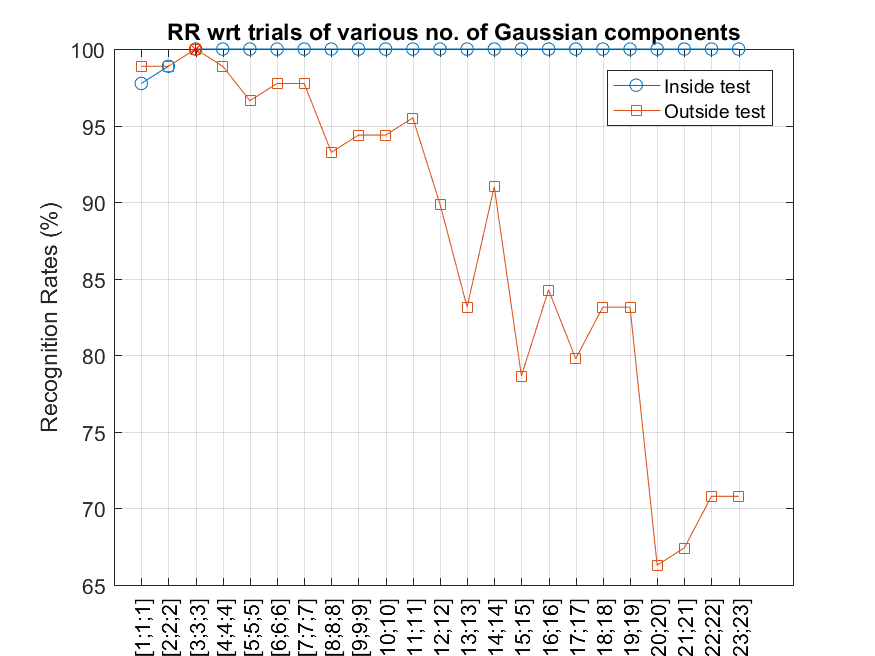In order to perform more analysis, we plot the range of all features:

Example 7: rangePlotWine.m[DS, TS]=prData('wine'); dsRangePlot(DS);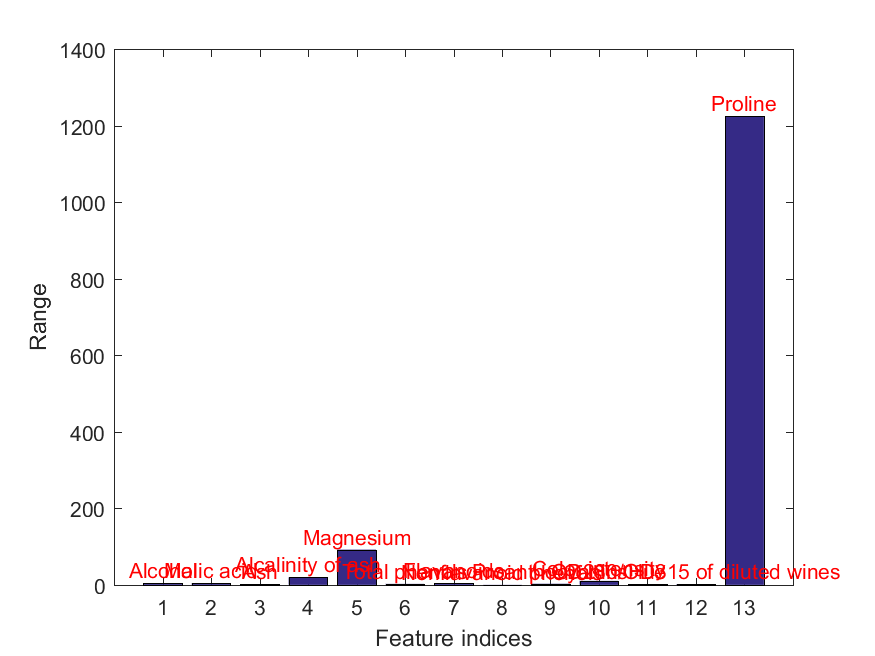Obviously the last feature has a much wider range than the others. We can perform input normalization before GMM training, as follows:

Example 8: gmmcWine03.m[DS, TS]=prData('wine'); [DS.input, mu, sigma]=inputNormalize(DS.input); % Input normalization for DS TS.input=inputNormalize(TS.input, mu, sigma); % Input normalization for TS count1=dsClassSize(DS); count2=dsClassSize(TS); gmmcOpt=gmmcTrain('defaultOpt'); gmmcOpt.arch.gaussianNum=1:min([count1, count2]); plotOpt=1; [gmmData, recogRate1, recogRate2]=gmmcGaussianNumEstimate(DS, TS, gmmcOpt, plotOpt); DS data count = 89, TS data count = 89 DS class data count = [29 36 24] TS class data count = [30 35 24] 1/24: No. of Gaussian = [1;1;1] ===> inside RR = 97.7528%, outside RR = 98.8764% 2/24: No. of Gaussian = [2;2;2] ===> inside RR = 98.8764%, outside RR = 98.8764% 3/24: No. of Gaussian = [3;3;3] ===> inside RR = 100%, outside RR = 98.8764% 4/24: No. of Gaussian = [4;4;4] ===> inside RR = 100%, outside RR = 100% 5/24: No. of Gaussian = [5;5;5] ===> inside RR = 100%, outside RR = 98.8764% 6/24: No. of Gaussian = [6;6;6] ===> inside RR = 100%, outside RR = 95.5056% 7/24: No. of Gaussian = [7;7;7] ===> inside RR = 100%, outside RR = 96.6292% 8/24: No. of Gaussian = [8;8;8] ===> inside RR = 100%, outside RR = 85.3933% 9/24: No. of Gaussian = [9;9;9] ===> inside RR = 100%, outside RR = 86.5169% 10/24: No. of Gaussian = [10;10;10] ===> inside RR = 100%, outside RR = 83.1461% 11/24: No. of Gaussian = [11;11;11] ===> inside RR = 100%, outside RR = 87.6404% 12/24: No. of Gaussian = [12;12;12] ===> inside RR = 100%, outside RR = 88.764% 13/24: No. of Gaussian = [13;13;13] ===> inside RR = 100%, outside RR = 88.764% 14/24: No. of Gaussian = [14;14;14] ===> inside RR = 100%, outside RR = 91.0112% 15/24: No. of Gaussian = [15;15;15] ===> inside RR = 100%, outside RR = 78.6517% 16/24: No. of Gaussian = [16;16;16] ===> inside RR = 100%, outside RR = 64.0449% 17/24: No. of Gaussian = [17;17;17] ===> inside RR = 100%, outside RR = 74.1573% 18/24: No. of Gaussian = [18;18;18] ===> inside RR = 100%, outside RR = 65.1685% 19/24: No. of Gaussian = [19;19;19] ===> inside RR = 100%, outside RR = 65.1685% 20/24: No. of Gaussian = [20;20;20] ===> inside RR = 100%, outside RR = 57.3034% 21/24: No. of Gaussian = [21;21;21] ===> inside RR = 100%, outside RR = 57.3034% 22/24: No. of Gaussian = [22;22;22] ===> inside RR = 100%, outside RR = 56.1798% 23/24: No. of Gaussian = [23;23;23] ===> inside RR = 100%, outside RR = 56.1798% 24/24: No. of Gaussian = [24;24;24] ===> Error out on errorTrialIndex=24 and errorClassIndex=3 [Warning: Using an integer to specify the legend location is not supported. Specify the legend location with respect to the axes using the 'Location' parameter.] [> In <a href="matlab:matlab.internal.language.introspective.errorDocCallback('legend>process_inputs', 'C:\Program Files\MATLAB\R2016a\toolbox\matlab\scribe\legend.p', 499)" style="font-weight:bold">legend>process_inputs</a> (<a href="matlab: opentoline('C:\Program Files\MATLAB\R2016a\toolbox\matlab\scribe\legend.p',499,0)">line 499</a>) In <a href="matlab:matlab.internal.language.introspective.errorDocCallback('legend>make_legend', 'C:\Program Files\MATLAB\R2016a\toolbox\matlab\scribe\legend.p', 303)" style="font-weight:bold">legend>make_legend</a> (<a href="matlab: opentoline('C:\Program Files\MATLAB\R2016a\toolbox\matlab\scribe\legend.p',303,0)">line 303</a>) In <a href="matlab:matlab.internal.language.introspective.errorDocCallback('legend', 'C:\Program Files\MATLAB\R2016a\toolbox\matlab\scribe\legend.p', 254)" style="font-weight:bold">legend</a> (<a href="matlab: opentoline('C:\Program Files\MATLAB\R2016a\toolbox\matlab\scribe\legend.p',254,0)">line 254</a>) In <a href="matlab:matlab.internal.language.introspective.errorDocCallback('gmmcGaussianNumEstimate', 'd:\users\jang\matlab\toolbox\machineLearning\gmmcGaussianNumEstimate.m', 123)" style="font-weight:bold">gmmcGaussianNumEstimate</a> (<a href="matlab: opentoline('d:\users\jang\matlab\toolbox\machineLearning\gmmcGaussianNumEstimate.m',123,0)">line 123</a>) In <a href="matlab:matlab.internal.language.introspective.errorDocCallback('gmmcWine03', 'd:\users\jang\books\dcpr\example\gmmcWine03.m', 8)" style="font-weight:bold">gmmcWine03</a> (<a href="matlab: opentoline('d:\users\jang\books\dcpr\example\gmmcWine03.m',8,0)">line 8</a>) In <a href="matlab:matlab.internal.language.introspective.errorDocCallback('goWriteOutputFile>dummyFunction', 'd:\users\jang\books\goWriteOutputFile.m', 85)" style="font-weight:bold">goWriteOutputFile>dummyFunction</a> (<a href="matlab: opentoline('d:\users\jang\books\goWriteOutputFile.m',85,0)">line 85</a>) In <a href="matlab:matlab.internal.language.introspective.errorDocCallback('goWriteOutputFile', 'd:\users\jang\books\goWriteOutputFile.m', 55)" style="font-weight:bold">goWriteOutputFile</a> (<a href="matlab: opentoline('d:\users\jang\books\goWriteOutputFile.m',55,0)">line 55</a>)]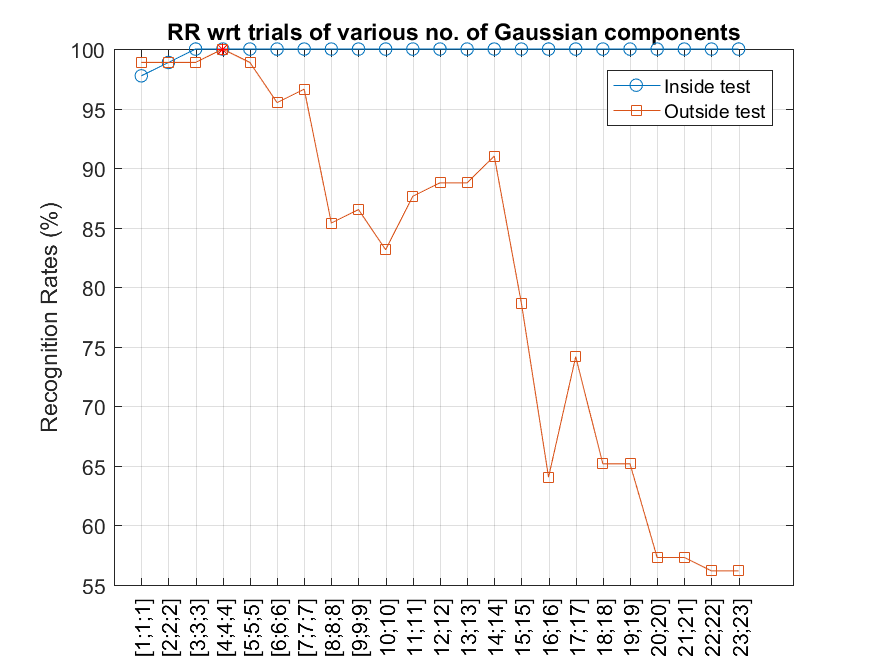The above plot demonstrates that input normalization can sometimes lead to a better accuracy.

Data Clustering and Pattern Recognition (資料分群與樣式辨認)